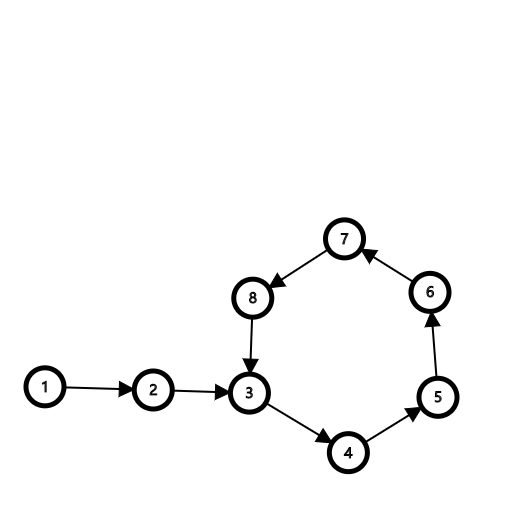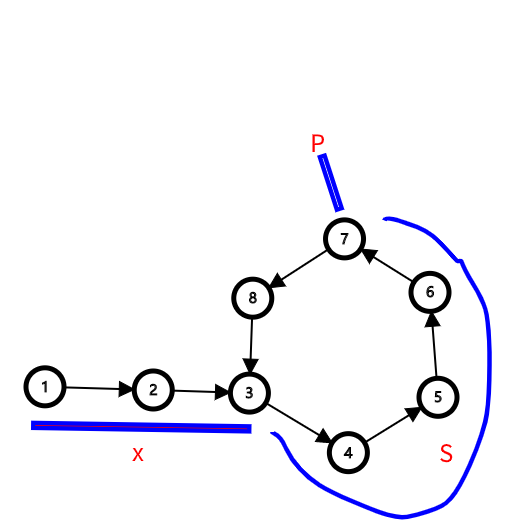slow 指针总共走了 $x+S$ 步，由于 fast 指针速度为 slow 指针2倍，所以 fast 指针走了$2x+2S$ 步。

slow 指针在环中走了 $L(slow)=S$ 步，fast 指针在环中走了 $L(fast)=x+2S$ 步。

$L(fast)-L(slow)=(x+2S)-S=x+S>S$

$S\geq n$，则 $L(fast)-L(slow)>n$$x+S=n------------------------①$

slow 指针走到环的入口处需要 $x$ 步，故当 slow 指针在环的入口处时，fast 指针距离环的入口 $S+x$ 步（fast 指针步长已经被调为1)，